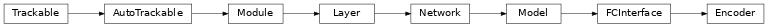Encoder¶

Inheritance Diagramclass ashpy.models.fc.encoders.Encoder(hidden_units, output_shape)[source]

Primitive Model for all fully connected encoder based architecture.

Examples

encoder = Encoder(
hidden_units=[256,128,64],
output_shape=10)
print(encoder(tf.zeros((1,55))).shape)
(1, 10)

Methods

 __init__(hidden_units, output_shape) Instantiate the Decoder.

Attributes

 activity_regularizer Optional regularizer function for the output of this layer. dtype dynamic inbound_nodes Deprecated, do NOT use! Only for compatibility with external Keras. input Retrieves the input tensor(s) of a layer. input_mask Retrieves the input mask tensor(s) of a layer. input_shape Retrieves the input shape(s) of a layer. input_spec Gets the network’s input specs. layers losses Losses which are associated with this Layer. metrics Returns the model’s metrics added using compile, add_metric APIs. metrics_names Returns the model’s display labels for all outputs. name Returns the name of this module as passed or determined in the ctor. name_scope Returns a tf.name_scope instance for this class. non_trainable_variables non_trainable_weights outbound_nodes Deprecated, do NOT use! Only for compatibility with external Keras. output Retrieves the output tensor(s) of a layer. output_mask Retrieves the output mask tensor(s) of a layer. output_shape Retrieves the output shape(s) of a layer. run_eagerly Settable attribute indicating whether the model should run eagerly. sample_weights state_updates Returns the updates from all layers that are stateful. stateful submodules Sequence of all sub-modules. trainable trainable_variables Sequence of variables owned by this module and it’s submodules. trainable_weights updates variables Returns the list of all layer variables/weights. weights Returns the list of all layer variables/weights.
__init__(hidden_units, output_shape)[source]

Instantiate the Decoder.

Parameters: hidden_units (tuple of int) – Number of units per hidden layer. output_shape (int) – Amount of units of the last tf.keras.layers.Dense. None